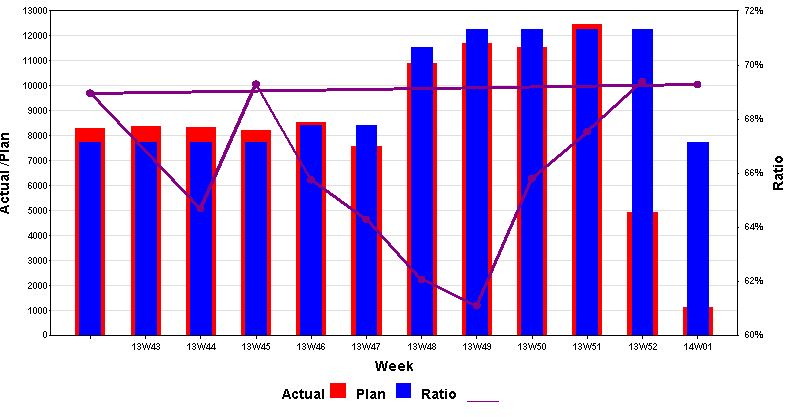## SAS 9.1 proc gplot order axis

Hi can somebody help mi with this.

My question is how to put two dimension  on horizontal axis wk and year to get proper order from wk 42/2013 to 1/2014.

This script was fine until first week of 2014.

I tried to make new variable with compress (year|| "*"||wk)  and then to split axis by * but there was error : All variables must be the same type for overlay request.

 data pass_fail;

 input wk actual pass fail year; datalines; 1 423 423 0 2014 42 6929 6915 14 2013 43 9344 9334 10 2013 44 7329 7322 7 2013 45 8263 8248 15 2013 46 8266 8252 14 2013 47 7525 7520 5 2013 48 10299 10276 23 2013 49 11059 11056 3 2013 50 10444 10443 1 2013 51 11776 11776 0 2013 52 5015 5014 1 2013

;

run;

data plan;

 set pass_fail; plan = 8400; format ratio percent7.; ratio = pass / (pass + fail ) ; week=wk-.13;

run;

goptions reset=ALL gsfmode=replace htext=30pt cback=white border htitle=32pt htext=16pt ftext="Arial/bold";

axis1 label=(a=90 h=25pt 'Actual /Plan') order=(0 to 12000 by 2000) minor=none offset=(0,0);

axis2 label=(a=90 h=25pt'Ratio') order=(.82 to 1.00 by .01) minor=none offset=(0,0);

axis3 label=( h=25pt'Week') minor=none offset=(0,10) value=(t=1 ' ');

symbol1 value=dot cv=purple height=2 interpol=join width=5 color=purple ;

symbol2 value=none interpol=needle width=50 color=red;

symbol3 value=none interpol=needle width=50 color=blue;

footnote h = 25pt c = black 'Actual ' c=Red f=marker 'U'

 h = 25pt  c=black f='Arial/bold' '  Plan  ' c=blue  f=marker 'U' h = 25pt  c=black f='Arial/bold' '  Ratio  ' c=purple   '____';

proc gplot data=plan;

plot actual*wk=2 plan*week=3 / overlay

vzero vaxis=axis1 haxis=axis3 autovref cvref=graydd;

plot2 ratio*wk=1 /  vaxis=axis2 ;

run;

quit;

1 ACCEPTED SOLUTION

Accepted Solutions

## Re: SAS 9.1 proc gplot order axis

Gplot doesn't support a 2-level axis (like a grouped bar chart, with year & week).

In your case, I'd recommend using real SAS 'date' values, and applying a 'week' format (such as weeku, weekv, or weekw).  Here's a page describing thesw formats:

http://support.sas.com/documentation/cdl/en/lrdict/64316/HTML/default/viewer.htm#a002604217.htm

7 REPLIES 7

## Re: SAS 9.1 proc gplot order axis

Gplot doesn't support a 2-level axis (like a grouped bar chart, with year & week).

In your case, I'd recommend using real SAS 'date' values, and applying a 'week' format (such as weeku, weekv, or weekw).  Here's a page describing thesw formats:

http://support.sas.com/documentation/cdl/en/lrdict/64316/HTML/default/viewer.htm#a002604217.htm

Hi Robert

Thanks again.

## Re: SAS 9.1 proc gplot order axis

Rpbert

Just one more question. As you can see on image there is two lines for Ratio.

In previous example I used week = wk - ..13; to avoid this.

This was worked because wk was formated as numeric. Now I use  different format, weekv5., which not allow me to do the same.

proc gplot data=plan;

plot actual*wk=2 plan*week=3 / overlay

vzero vaxis=axis1 haxis=axis3 autovref cvref=graydd;

plot2 ratio*wk=1 /  vaxis=axis2 ;

run;Is there any way how to fix this and get proper result?

## Re: SAS 9.1 proc gplot order axis

The underlying date values are still numeric, so you can still use the "subtract an offset" technique to get one of the needle-bars to move to the left. But the offset will have to be a much larger number now - experiment with it a little, and see what offset works best for your situation.

## Re: SAS 9.1 proc gplot order axis

My problem is in two RATIO lines. It should be just one line showing ratio for period 3013/2014 .

Now I have one line for each year. Not sure where the problem is.

Thanks

## Re: SAS 9.1 proc gplot order axis

Ahh - now I understand.

I think you'll need to sort your data by the date variable. Since you have the data from the 2nd year first in your data, it starts drawing the line there, and then when it gets to the data from the first year it then connects the points on the right of the graph to the points back on the left of the graph (creating the extra/stray line across the graph). Gplot simply connects the points in the order they are in the data.